Let us follow the table given below: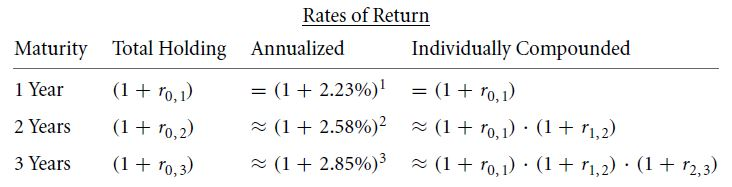Let us refer to our discussion of yield curve and recall the values. Substituting those values, we get: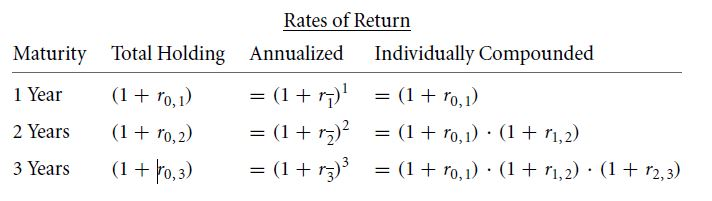Calculating the holding rates of interest in the second column, we get: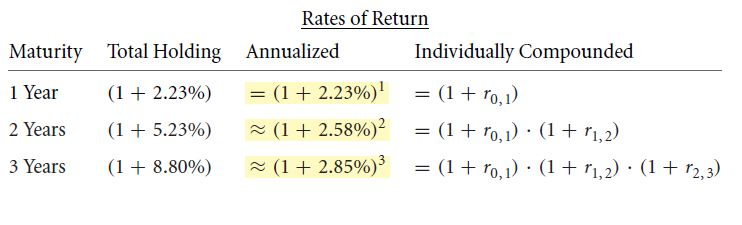We know r 0, 1=2.23%. In the same way, the implied future rates can also be calculated: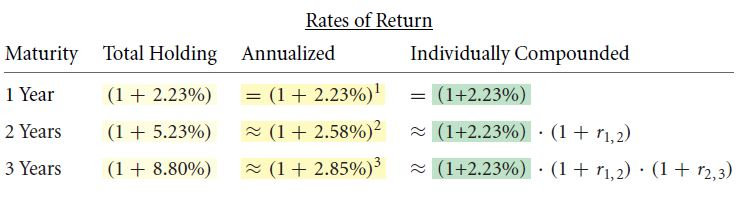r1, 2 and r 2,3can be determined as: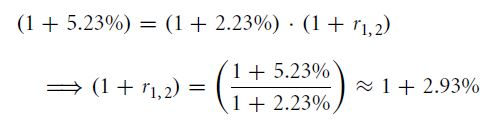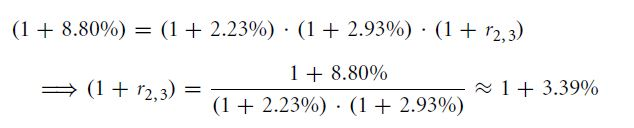Putting these values in the table, we get: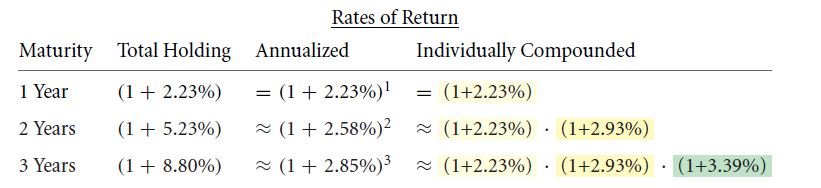As all the forward interest rates have been obtained, the yield curve can be plotted. The annualized interest rate is the geometric average of all the years taken into the calculation. If we know the average interest rate and the first half of the average, then the second half can be calculated.

Links of Next Financial Accounting Topics:-### Customer Reviews

My Homework Help
Rated 5.0 out of 5 based on 510 customer reviews at